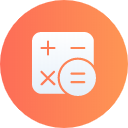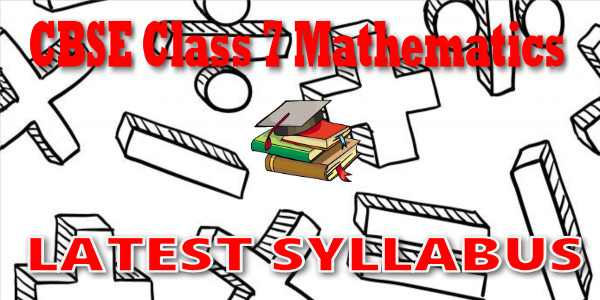No products in the cart.

# CBSE - Class 07 - Mathematics - CBSE Syllabus## CBSE, JEE, NEET, CUET

Question Bank, Mock Tests, Exam Papers

NCERT Solutions, Sample Papers, Notes, Videos

## CBSE Syllabus for Class 07 Mathematics

CBSE Syllabus Class 7 Mathematics### Student Subscription

##### Unlock the exclusive content designed for the toppers## myCBSEguide App

Complete Guide for CBSE Students

NCERT Solutions, NCERT Exemplars, Revison Notes, Free Videos, CBSE Papers, MCQ Tests & more.

# Latest CBSE Syllabus for Class 7 MathematicsCBSE syllabus for class 7 Mathematics 2018, 2019, 2020, 2021, 2022, 2023 as per cbse.nic.in new curriculum. CBSE syllabus is available for free download in PDF format. Download latest CBSE syllabus of 7th Mathematics as PDF format. Chemisty syllabus for cbse class 7 is also available in myCBSEguide app, the best app for CBSE students.

## CBSE Academics Unit - Curriculum Syllabus

CBSE has special academics unit to design curriculum and syllabus. The syllabus for CBSE class 7 Mathematics is published by cbse.nic.in Central Secondary Education, Head Office in New Delhi. The latest syllabus for class 7 Mathematics includes list of topics and chapters in Mathematics. CBSE question papers are designed as per the syllabus prescribed for current session.

## CBSE Syllabus category

• Secondary School Curriculum (class 9 and class 10)
• Senior School Curriculum (class 11 and class 12)
• Vocational Courses (Class 11 and class 12)

Course Structure for Class-VII
Number System (50 hrs.)
(i) Knowing our Numbers: Integers
• Multiplication and division of integers (through patterns). Division by zero is meaningless
• Properties of integers (including identities for addition & multiplication, commutative, associative, distributive) (through patterns). These would include examples from whole numbers as well. Involve expressing commutative and associative properties in a general form. Construction of counterexamples, including some by children. Counter examples like
• Subtraction is not commutative.
• Word problems including integers (all operations)

(ii) Fractions and rational numbers:
• Multiplication of fractions
• Fraction as an operator
• Reciprocal of a fraction
• Division of fractions
• Word problems involving mixed fractions
• Introduction to rational numbers (with representation on number line)
• Operations on rational numbers (all operations)
• Representation of rational number as a decimal.
• Word problems on rational numbers (all operations)
• Multiplication and division of decimal fractions
• Conversion of units (length & mass)
• Word problems (including all operations)

Algebra (20 hrs.)
ALGEBRAIC EXPRESSIONS
• Generate algebraic expressions (simple) involving one or two variables
• Identifying constants, coefficient, powers
• Like and unlike terms, degree of expressions e.g., x2y etc. (exponent≤3, number of variables)
• Addition, subtraction of algebraic expressions (coefficients should be integers).
• Simple linear equations in one variable (in contextual problems) with two operations (avoid complicated coefficients)

Ratio and Proportion (20 hrs.)
• Ratio and proportion (revision)
• Unitary method continued, consolidation, general expression.
• Percentage- an introduction.
• Understanding percentage as a fraction with denominator 100
• Converting fractions and decimals into percentage and vice-versa.
• Application to profit and loss (single transaction only)
• Application to simple interest (time period in complete years).

Geometry (60 hrs.)
(i) Understanding shapes:
• Pairs of angles (linear, supplementary, complementary, adjacent, vertically opposite) (verification and simple proof of vertically opposite angles)
• Properties of parallel lines with transversal (alternate, corresponding, interior, exterior angles)

(ii) Properties of triangles:
• Angle sum property (with notions of proof & verification through paper folding, proofs using property of parallel lines, difference between proof and verification.)
• Exterior angle property
• Sum of two sides of a its third side
• Pythagoras Theorem (Verification only)

(iii) Symmetry
• Recalling reflection symmetry
• Idea of rotational symmetry, observations of rotational symmetry of 2-D objects. (900, 1200, 1800)
• Operation of rotation through 900 and 1800 of simple figures.
• Examples of figures with both rotation and reflection symmetry (both operations)
• Examples of figures that have reflection and rotation symmetry and vice-versa

(iv) Representing 3-D in 2-D:
• Drawing 3-D figures in 2-D showing hidden faces.
• Identification and counting of vertices, edges, faces, nets (for cubes cuboids, and cylinders, cones). • Matching pictures with objects (Identifying names)
• Mapping the space around approximately through visual estimation.

(v) Congruence
• Congruence through superposition (examples-blades, stamps, etc.)
• Extend congruence to simple geometrical shapes e.g. triangles, circles.
• Criteria of congruence (by verification) SSS, SAS, ASA, RHS

(vi) Construction (Using scale, protractor, compass)
• Construction of a line parallel to a given line from a point outside it. (Simple proof as remark with the reasoning of alternate angles)

• Construction of simple triangles. Like given three sides, given a side and two angles on it, given two sides and the angle between them.

Mensuration (15 hrs.)
• Revision of perimeter, Circumference of Circle

Area
Concept of measurement using a basic unit area of a square, rectangle, triangle, parallelogram and circle, area between two rectangles and two concentric circles.

Data handling (15 hrs.)
i. Collection and organization of data – choosing the data to collect for a hypothesis testing.
ii. Mean, median and mode of ungrouped data – understanding what they represent.
iii. Constructing barographs
iv. Feel of probability using data through experiments. Notion of chance in events like tossing coins, dice etc. Tabulating and counting occurrences of 1 through 6 in a number of
v. throws. Comparing the observation with that for a coin. Observing strings of throws, notion of randomness.

## CURRICULUM / SYLLABUS OF VOCATIONAL COURSES

CURRICULUM / SYLLABUS

 Scheme of Studies and List of Vocational Courses Application form to offer vocational courses Secondary School Curriculum (Under NSQF) Senior School Curriculum (Vocational)## myCBSEguide

Trusted by 1 Crore+ Students## Test Generator

Create papers online. It's FREE.## CUET Mock Tests

75,000+ questions to practice only on myCBSEguide app